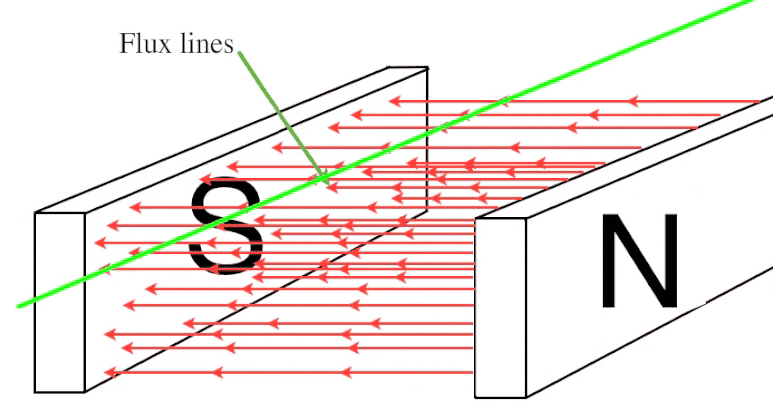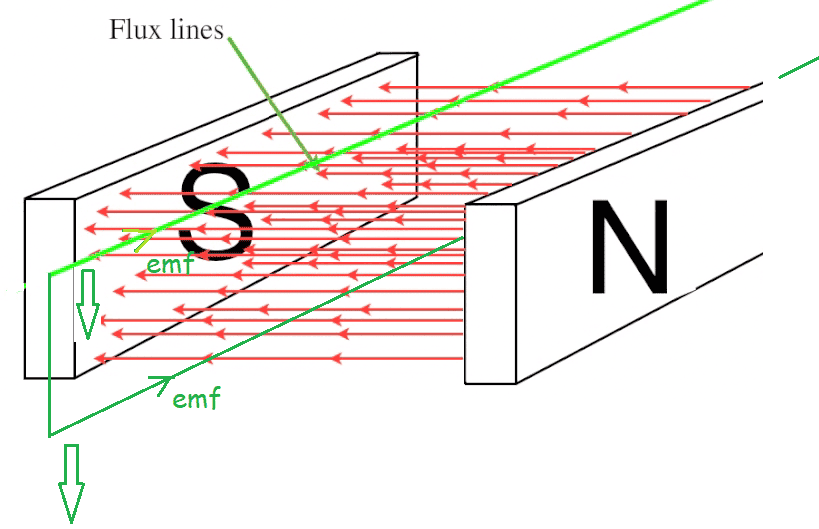# The EMF induced in straight current-carrying conductor

funmi
Why is an emf induced in straight current carrying conductor as it moves at at right angles to a uniform and constant magnetic filed. By Faraday's law, this e.m.f. is equal to dΦ/dt but I do not understand how a wire cutting a uniform magnetic field experiences a change in magnetic flux . Its area is constant and magnetic flux density is constant so the magnetic flux felt by the wire Φ=BA is constant.Wire(green) is moving down at right angles to the field

Last edited:

Homework Helper

And indicate the direction of motion of the wire in the drawing

•berkeman
Faraday's law can be written as:
emf = d/dt BAN
Assume B is constant and N =1 we can write:
emf = B dA/dt
In this example dA/dt is the rate of change of area in other words the area sliced out by the wire in one second. If the wire has length l and is moving up or down with velocity v the area sliced out per second will be lv. Make a sketch and hopefully you will see it.

funmi
Faraday's law can be written as:
emf = d/dt BAN
Assume B is constant and N =1 we can write:
emf = B dA/dt
In this example dA/dt is the rate of change of area in other words the area sliced out by the wire in one second. If the wire has length l and is moving up or down with velocity v the area sliced out per second will be lv. Make a sketch and hopefully you will see it.
but this doesn't work for a coil, if i replaced the straight wire with a coil already in the magnetic field and moved it the same way with no rotation no emf would be induced. why is it different for a coil and a straight current carrying conductor

Homework Helper
Gold Member
Perhaps because the coil has two sides and the emf in each side opposes when they both move in the same direction.Mentor
By Faraday's law, this e.m.f. is equal to dΦ/dt but I do not understand how a wire cutting a uniform magnetic field experiences a change in magnetic flux . Its area is constant and magnetic flux density is constant so the magnetic flux felt by the wire Φ=BA is constant.
Well, there isn’t really a loop here, so Faraday’s law doesn’t really make a difference in this case. If you make the “loop” with zero area by going backwards and forwards along the same line then by Faraday’s law you get 0 EMF and any voltage distribution along the wire is consistent with 0 EMF since you will go forwards and backwards across the same distribution. So basically, you need to look for a different law.

The law to look at is the Lorentz force law.

if i replaced the straight wire with a coil already in the magnetic field and moved it the same way with no rotation no emf would be induced.
That is correct. No EMF is induced in such a coil moving through a uniform magnetic field.

Last edited:
•funmi
For a closed loop moving at steady velocity within a uniform B field there will be no internal current but, as predicted by the Lorentz law, there will be a build up of electrons, most concentrated at one end of the loop, and a shortage of electrons, most concentrated at the other end. Hence there will be an emf. A current can be drawn by adding external circuitry eg a piece of wire connected to the ends of the loop.

•funmi
Mentor
Hence there will be an emf.
Not around the whole loop. The EMF on one side cancels out the EMF on the other for a total of 0 EMF around the loop.

•funmi
funmi
Well, there isn’t really a loop here, so Faraday’s law doesn’t really make a difference in this case. If you make the “loop” with zero area by going backwards and forwards along the same line then by Faraday’s law you get 0 EMF and any voltage distribution along the wire is consistent with 0 EMF since you will go forwards and backwards across the same distribution. So basically, you need to look for a different law.

The law to look at is the Lorentz force law.

That is correct. No EMF is induced in such a coil moving through a uniform magnetic field.
Thank you I think I understand, just to clarify I can make a "loop" while moving the wire forward and backward( to make the loop) as I move it up and down between the magnets, it will produce no emf because the direction of the current will be the same as wire's movement whether forward or backward.

funmi
For a closed loop moving at steady velocity within a uniform B field there will be no internal current but, as predicted by the Lorentz law, there will be a build up of electrons, most concentrated at one end of the loop, and a shortage of electrons, most concentrated at the other end. Hence there will be an emf. A current can be drawn by adding external circuitry eg a piece of wire connected to the ends of the loop.
Thanks

Mentor
I can make a "loop" while moving the wire forward and backward( to make the loop)
Not exactly. In this context a "loop" is a closed path at a given moment in time. So if you have to spend time to make a closed path then it isn't a "loop" in the meaning of this law.

There are other contexts where the sort of loop you are describing is perfectly acceptable, such as describing the work done on a point particle moving in a conservative force field, but in Maxwell's equations the surface and loop integrals are at a given instant of time. Those loops can change over time, either by stretching or moving, but at every moment they are a complete closed path.